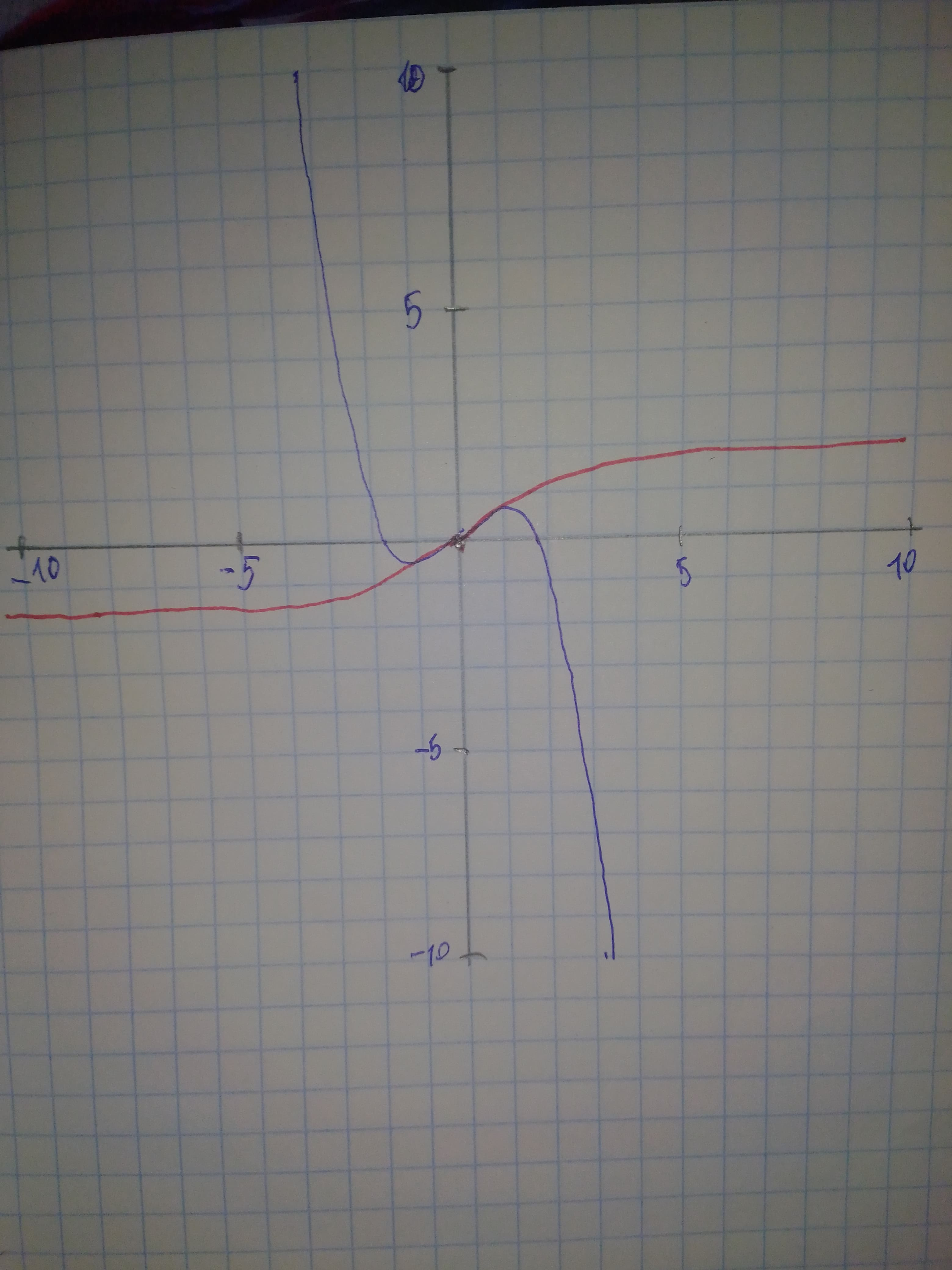# (a) find the Maclaurin polynomial P_{3}(x) for f(x), (b) complete the followingDottie Parra 2021-02-02 Answered

(a) find the Maclaurin polynomial ${P}_{3}\left(x\right)$ for f(x), (b) complete the following

$\begin{array}{|cccccccc|}\hline x:& -0.75& -0.50& -0.25& 0& 0.25& 0.50& 0.75\\ \hline\end{array}$

for f(x) and ${P}_{3}\left(x\right)$ and (c) sketch the graphs of f(x) and ${P}_{3}\left(x\right)$ on the same set of coordinate axes. $f\left(x\right)=\mathrm{arctan}x$

You can still ask an expert for help

• Questions are typically answered in as fast as 30 minutes

Solve your problem for the price of one coffee

• Math expert for every subject
• Pay only if we can solve itAdnaan Franks

Step 1 a) $f\left(x\right)=\mathrm{arctan}x$
${P}_{3}\left(x\right)=\sum _{k=0}^{3}\frac{{f}^{\left(k\right)}\left(0\right)}{k!}{x}^{k}$
${P}_{3}\left(x\right)=x-\frac{1}{3}{x}^{3}$

Step 2 b)

$\begin{array}{|cccccccc|}\hline x& -0.75& -0.50& -0.25& -0.0& 0.25& 0.50& 0.75\\ f\left(x\right)& -0.6435& -0.4636& -0.2449& 0& 0.2449& 0.4636& 0.6435\\ {P}_{3}\left(x\right)& -0.6093& -0.4583& -0.2447& 0& 0.2447& 0.4583& 0.6093\\ \hline\end{array}$

On the graph below: Red curve = $f\left(x\right)$ Blue curve = ${P}_{3}\left(x\right)$ Step 3